# Exercise E6.1 Lines and Angles NCERT Solutions Class 9

Go back to  'Lines and Angles'

## Chapter 6 Ex.6.1 Question 1

In the given figure lines $$AB$$ and $$CD$$ intersect at $$O.$$ If  \begin{align} \angle AOC + \angle BOE = 70 ^ { \circ } \end{align} and \begin{align} \angle BOD = 40 ^ { \circ }, \end{align} find $$\angle BOE$$ and reflex $$\angle COE$$.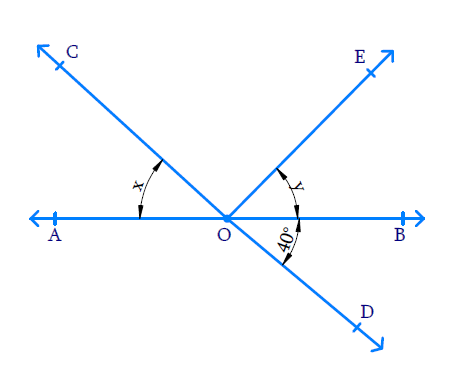### Solution

What is known?

$$\angle AOC+\angle BOE={70}^{\circ}$$ and $$\angle BOD = 40^{\circ}$$

What is unknown?

$$\angle BOE =?,$$ Reflex $$\angle COE =?$$

Reasoning:

We know that vertically opposite angles formed when two lines intersect are equal. Also, sum of the adjacent angles is $$180$$ degrees.

Steps:

Let $$\Delta AOC = \rm x$$ and $$\rm{}\Delta BOE =\rm y.$$

Then $$x+ y=70^\circ$$ $$(\because AOC+BOE=70^\circ )$$

Let Reflex $$\Delta COE = \rm z$$

We can see that $$AB$$ and $$CD$$ are two intersecting lines so the pair of angles formed are vertically opposite angles and they are equal.

$$\text{i.e. } \angle AOD$$ and $$\angle BOC$$ and $$\angle AOC = \angle BOD.$$

Since $$\angle AOC = x$$  and $$\angle AOC = \angle BOD = 40^ {\circ},$$

we can say that  $$x = 40^ {\circ}.$$

Also we know that,

\begin{align} x + y &= 70 ^ {\circ } \\ 40 ^ { \circ } + y &= 70 ^ {\circ } \\ y &= 70 ^ { \circ } - 40 ^ {\circ } \\ y &= 30 ^ { \circ } \\ \angle BOE &= 30 ^ { \circ } \end{align}

If we consider line $$AB$$ and ray $$OD$$ on it, then $$\angle AOD$$ and $$\angle BOD$$ are adjacent angles.

\begin{align} \angle AOD + \angle BOD &= 180 ^ { \circ } \\ \angle AOD + 40 ^ { \circ } &= 180 ^ { \circ } \\ \angle AOD &= 180 ^ { \circ } - 40 ^ { \circ } \\ &= 140 ^ { \circ } \end{align}

\begin{align}\text{Reflex }\angle COE &=\begin{pmatrix}\angle AOC +\\ \angle AOD +\\ \angle BOD +\\ \angle BOE \end{pmatrix} \\ &= \begin{pmatrix}40 ^ { \circ } + 140 ^ { \circ } \\+ 40 ^ { \circ } + 30 ^ { \circ } \end{pmatrix}\\ &= 250 ^ { \circ } \end{align}

## Chapter 6 Ex.6.1 Question 2

In the given figure, lines $$XY$$ and $$MN$$ intersect at $$O.$$ If $$\angle POY = 90^ {\circ}$$ and $$a:b = 2:3,$$ find $$c.$$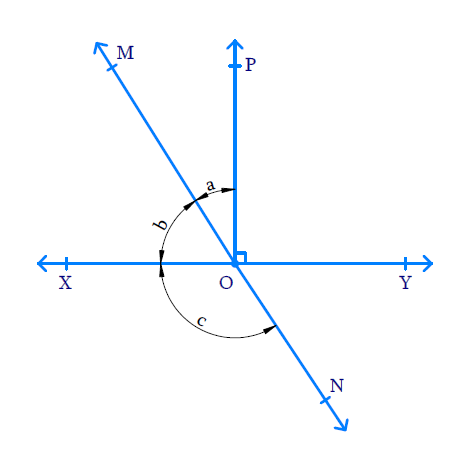### Solution

What is known?

$$\angle POY= 90^ {\circ}$$ and $$a:b = 2:3$$

What is unknown?

$$\angle XON = c =?$$

Reasoning:

If two lines intersect each other, then the vertically opposite angles formed are equal.

Steps:

Line $$OP$$ is perpendicular to line $$XY.$$ Hence $$\angle POY = \angle POX = 90^ {\circ}$$

\begin{align}\angle POX & = \angle POM + \angle MOX\end{align}

\begin{align} 90 ^ { \circ } & = a + b \quad \ldots \ldots . ( 1 ) \end{align}

Since $$a$$ and $$b$$ are in the ratio $$2:3$$ that is, $$a = 2x$$ and $$b = 3x \quad \ldots \ldots . (2)$$

Substituting ($$2$$) in ($$1$$),

\begin{align} a + b &= 90 ^ { \circ } \\ 2 x + 3 x &= 90 ^ { \circ } \\ 5 x &= 90 ^ { \circ } \\ x = \frac { 90 } { 5 } &= 18 ^ { \circ } \\ a = 2 x &= 2 \times 18 ^ { \circ } \\ a &= 36 ^ { \circ } \\ b = 3 x &= 3 \times 18 ^ { \circ } \\ b &= 54 ^ { \circ } \end{align}

Also,\begin{align}\angle MOY & = \angle MOP+ \angle POY\end{align}

\begin{align} & = a + 90 ^ { \circ } \\ & = 36 ^ { \circ } + 90 ^ { \circ } \\&= 126 ^ { \circ } \end{align}

Lines $$MN$$ and $$XY$$ intersect at point $$O$$ and the vertically opposite angles formed are equal.

\begin{align}\angle XON &= \angle MOY \\ c &=126^ {\circ} \end{align}

## Chapter 6 Ex.6.1 Question 3

In the given figure, $$\angle PQR = \angle PRQ$$ then prove that $$\angle PQS = \angle PRT.$$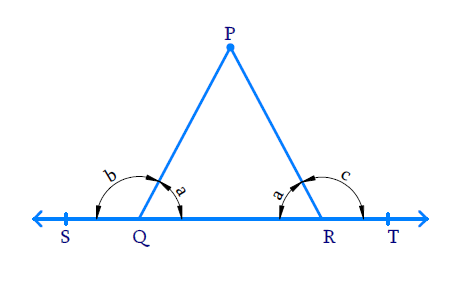### Solution

What is known?

$$\angle PQR = \angle PRQ$$

What is unknown?

To prove $$\angle PQS = \angle PRT$$

Reasoning:

If a ray stands on a line, then the sum of adjacent angles formed is $$180^ {\circ}.$$

steps:

Let $$\angle PQR= \angle PRQ = a$$

Let $$\angle PQS = b$$ and $$\angle PRT = c$$

Line $$ST$$ and $$PQ$$ intersect at point $$Q$$, then the sum of adjacent angles $$\angle PQS$$ and $$\angle PQR$$ is $$180^ {\circ}.$$

\begin{align} \angle PQS + \angle PQR & = 180 ^ { \circ } \\ b + a & = 180 ^ { \circ } \\ b & = 180 ^ { \circ } - a\ldots . ( 1 ) \end{align}

Line $$ST$$ and $$PR$$ intersect at point $$R$$, then the sum of adjacent angles $$\angle PRQ$$ and $$\angle PRT$$ is $$180^ {\circ}.$$

\begin{align} \angle PRQ + \angle PRT & = 180 ^ { \circ } \\ a + c & = 180 ^ { \circ } \\ c & = 180 ^ { \circ } - a\ldots . ( 2 ) \end{align}

From equations ($$1$$) and ($$2$$), it is clear that $$b = c.$$

Hence $$\angle PQS = \angle PRT$$ is proved.

## Chapter 6 Ex.6.1 Question 4

In the given figure, if $$x+y = w+z,$$ then prove that $$AOB$$ is a line.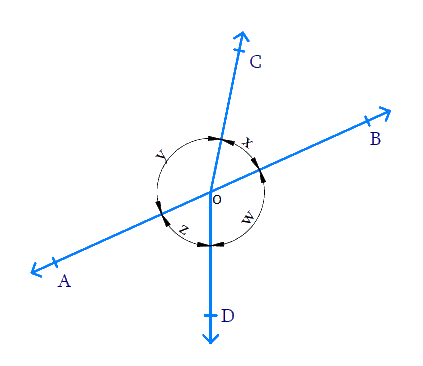### Solution

What is known?

\begin{align}x+y = w+z\end{align}

What is unknown?

To prove that $$AOB$$ is a line.

Reasoning:

If the sum of two adjacent angles is $$180^ {\circ}$$, then the non–common arms of the angles form a line.

Steps:

From the figure we can see that:

\begin{align}(x + y) +( w + z) = 360^ {\circ} \\ (\text {complete angle})\end{align}

It is given that $$(x + y) = (w + z),$$ Hence

$$(x + y) + (w + z) = 360^ {\circ}$$ can be written as $$(x + y) + (x +y) = 360^ {\circ}$$

\begin{align} 2 x + 2 y &= 360 ^ { \circ } \\ 2 ( x + y ) &= 360 ^ { \circ } \\ x + y &= \frac { 360 } { 2 } \\&= 180 ^ { \circ } \end{align}

Since sum of adjacent angles $$x$$ and $$y$$ with $$OA$$ and $$OB$$ as the non- common arms is $$180^ {\circ}$$ we can say that $$AOB$$ is a line.

## Chapter 6 Ex.6.1 Question 5

In the given figure, $$POQ$$ is a line. Ray $$OR$$ is perpendicular to line $$PQ.\;OS$$ another ray lying between rays $$OP$$ and $$OR.$$ Prove that \begin{align} \angle ROS =\frac {1}{2}(\angle QOS - \angle POS).\end{align}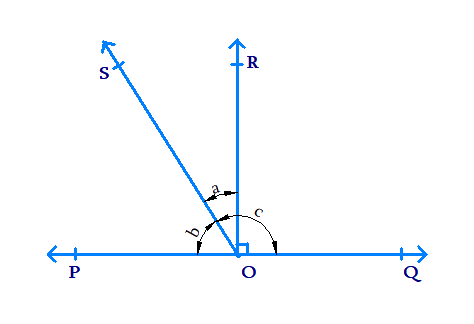### Solution

What is known?

$$OR$$ is perpendicular to $$PQ.$$ $$\angle ROQ = \angle ROP =90^ {\circ}.$$

What is unknown?

To prove that: \begin{align} \angle ROS =\frac {1}{2}(\angle QOS - \angle POS)\end{align}

Reasoning:

When a ray intersects a line, then the sum of adjacent angles so formed is $$180^ {\circ}.$$

Steps:

Let  $$\angle ROS = a, \angle POS = b$$  and $$\angle SOQ = c.$$

To prove that: \begin{align}a= \frac{1}{2}(c-b).\end{align}

Since $$\angle ROQ = \angle ROP = 90 ^ { \circ }$$

We can say,

\begin{align} \angle POS + \angle SOR &= \angle POR \end{align}

\begin{align}\rm{} b + a &= 90 ^ { \circ } \ldots \ldots \ldots ( 1 ) \end{align}

Line $$PQ$$ is intersected by ray $$OS.$$

Hence, \begin{align}\angle POS + \angle SOQ = \,& b + c = 180 ^ { \circ } \end{align}

\begin{align}& b + c = 180 ^ { \circ } \ldots \ldots \ldots (2) \end{align}

From equation (1), we get: $$a + b = 90^ {\circ}$$

Multiplying by $$2$$ on both sides we get,

\begin{align} 2 ( a + b ) &= 2 \times 90 ^ { \circ } \\ 2 ( a + b ) &= 180 ^ { \circ } \ldots \ldots \ldots ( 3 ) \end{align}

Comparing equations ($$3$$) and ($$2$$),

\begin{align} 2 ( a + b ) &= b + c \\ 2 a + 2 b &= b + c \\ 2 a &= b + c - 2 b \\ 2 a &= c - b \\ a &= \frac { 1 } { 2 } ( c - b ) \\ \therefore \angle ROS &= \frac { 1 } { 2 } ( \angle QOS - \angle POS). \end{align}

## Chapter 6 Ex.6.1 Question 6

It is given that $$\angle XYZ = 64^ {\circ}$$ and $$XY$$ is produced to point $$P.$$ Draw a figure from the given information. If ray $$YQ$$ bisects $$\angle ZYP,$$ find $$\angle XYQ$$ and reflex $$\angle QYP.$$

### Solution

What is known?

$$\angle XYZ = 64^ {\circ}$$ and Ray $$YQ$$ bisects $$\angle PYZ.$$

What is unknown?

$$\angle XYQ = ?$$ and Reflex $$\angle QYP = ?$$

Reasoning:

When a ray intersects a line sum of adjacent angles formed is $$180^ {\circ}.$$

Steps:

With the given information in the question, we can come up with this diagram.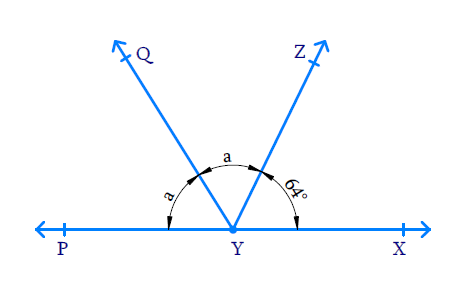Ray $$YQ$$ bisects $$\angle ZYP$$ and let $$\angle ZYQ = \angle QYP = a.$$

We can see from figure that $$PX$$ a line and $$YZ$$ is a ray intersecting at point $$Y$$ and the sum of adjacent angles so formed is $$180^ {\circ}.$$

Hence $$\angle ZYP + \angle ZYX = 180 ^ { \circ }$$

\begin{align}\angle ZYQ + \angle QYP + &\angle ZYX = 180 ^ { \circ } \\ a + a + 64 ^ {\circ } & = 180 ^ { \circ } \\ 2 a + 64 ^ {\circ } & = 180 ^ { \circ } \\ 2 a & = 180 ^ { \circ }-64 ^ {\circ } \\&= 116 ^ { \circ } \\ a & = \frac { 116 } { 2 } \\ & = 58 ^ { \circ } \end{align}

Then $$\angle XYQ = \angle XYZ + \angle ZYQ$$

\begin{align}\angle XYQ &= a + 64 ^ {\circ } \\ &= 58 ^ { \circ } + 64 ^ {\circ} \\ &= 122 ^ { 0 } \end{align}

As,

\begin{align}\angle QYP &= a, \text {Reflex}\angle QYP \\&= (360 ^ { \circ } - a) \end{align}

\begin{align}&= (360 ^ { \circ } - 58 ^ { \circ }) \\ &= 302 ^ { \circ } \end{align}

Reflex,$$\angle QYP = 302 ^ { \circ }$$

Related Sections
Related Sections
Instant doubt clearing with Cuemath Advanced Math Program